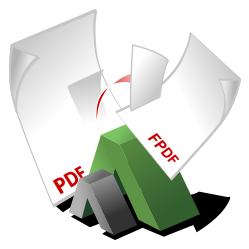# FPDI

## Demos of FPDI v1 (outdated)

This is the demos archive of FPDI v1.

### Simple Introduction Demo of FPDI

This demo simply imports a single page of an existing PDF document and adds text in front of it.

PHP
```<?php
require_once('fpdf.php');
require_once('fpdi.php');

// initiate FPDI
\$pdf = new FPDI();
// set the source file
\$pdf->setSourceFile('PdfDocument.pdf');
// import page 1
\$tplIdx = \$pdf->importPage(1);
// use the imported page and place it at position 10,10 with a width of 100 mm
\$pdf->useTemplate(\$tplIdx, 10, 10, 100);

// now write some text above the imported page
\$pdf->SetFont('Helvetica');
\$pdf->SetTextColor(255, 0, 0);
\$pdf->SetXY(30, 30);
\$pdf->Write(0, 'This is just a simple text');

\$pdf->Output();```

### Create Thumbnails of an existing PDF

This demo creates a thumbnail overview of an existing PDF document.

### Fake a concatenation of PDF documents

Yes, it's just a fake and not a real concatenation. As FPDI only imports single pages the structure of the resulting document is completely different from the one of the original. Also FPDI is not able to handle dynamic content like bookmark outlines, form fields, or any other annotation. These content get lost.

(For real concatenation see: SetaPDF-Merger component - this component works without modifying the internal structur of PDF documents and will keep all content.)

PHP
```<?php
require_once('fpdf.php');
require_once('fpdi.php');

class ConcatPdf extends FPDI
{
public \$files = array();

public function setFiles(\$files)
{
\$this->files = \$files;
}

public function concat()
{
foreach(\$this->files AS \$file) {
\$pageCount = \$this->setSourceFile(\$file);
for (\$pageNo = 1; \$pageNo <= \$pageCount; \$pageNo++) {
\$tplIdx = \$this->ImportPage(\$pageNo);
\$s = \$this->getTemplatesize(\$tplIdx);
\$this->AddPage(\$s['w'] > \$s['h'] ? 'L' : 'P', array(\$s['w'], \$s['h']));
\$this->useTemplate(\$tplIdx);
}
}
}
}

\$pdf = new ConcatPdf();
\$pdf->setFiles(array('Boombastic-Box.pdf', 'Fantastic-Speaker.pdf', 'Noisy-Tube.pdf'));
\$pdf->concat();

\$pdf->Output('concat.pdf', 'I');```

### Import different boxes from one document

This demo shows how to import different boundary boxes of a pdf page.

PHP
```<?php
require_once('fpdf.php');
require_once('fpdi.php');

// initiate FPDI
\$pdf = new FPDI();
\$pdf->SetFont('Arial', '', 10);
// set the sourcefile
\$pdf->setSourceFile('PdfDocument.pdf');

\$offsetTop = 15;

// import MediaBox from page 1
\$box = '/MediaBox';
\$pdf->Cell(60, 4, \$box);
\$tplIdx = \$pdf->importPage(1, \$box);
\$size = \$pdf->useTemplate(\$tplIdx, 10, \$offsetTop, 50);
\$pdf->Rect(10, \$offsetTop, \$size['w'], \$size['h']);

// re-import page ones CropBox
\$box = '/CropBox';
\$pdf->Cell(60, 4, \$box);
\$tplIdx = \$pdf->importPage(1, \$box);
\$size = \$pdf->useTemplate(\$tplIdx, 70, \$offsetTop, 50);
\$pdf->Rect(70, \$offsetTop, \$size['w'], \$size['h']);

// re-import page ones TrimBox
\$box = '/TrimBox';
\$pdf->Cell(60, 4, \$box);
\$tplIdx = \$pdf->importPage(1, \$box);
\$size = \$pdf->useTemplate(\$tplIdx, 130, \$offsetTop, 50);
\$pdf->Rect(130, \$offsetTop, \$size['w'], \$size['h']);

\$offsetTop = 100;
\$pdf->Ln(\$offsetTop - 15);

// re-import page ones BleedBox
\$box = '/BleedBox';
\$pdf->Cell(60, 4, \$box);
\$tplIdx = \$pdf->importPage(1, \$box);
\$size = \$pdf->useTemplate(\$tplIdx, 10, \$offsetTop, 50);
\$pdf->Rect(10, \$offsetTop, \$size['w'], \$size['h']);

// re-import page ones ArtBox
\$box = '/ArtBox';
\$pdf->Cell(60, 4, \$box);
\$tplIdx = \$pdf->importPage(1, \$box);
\$size = \$pdf->useTemplate(\$tplIdx, 70, \$offsetTop, 50);
\$pdf->Rect(70, \$offsetTop, \$size['w'], \$size['h']);

\$pdf->Output('newpdf.pdf', 'I');```

### FPDI and TCPDF

This demo is based on example008.php of the TCPDF package. We used FPDI to import a vector logo as a PDF page and place it into the header of every page.

PHP
```<?php
// just require TCPDF instead of FPDF
require_once('tcpdf.php');
require_once('fpdi.php');

class PDF extends FPDI
{
/**
* "Remembers" the template id of the imported page
*/
var \$_tplIdx;

/**
* Draw an imported PDF logo on every page
*/
{
if (is_null(\$this->_tplIdx)) {
\$this->setSourceFile('logo.pdf');
\$this->_tplIdx = \$this->importPage(1);
}
\$size = \$this->useTemplate(\$this->_tplIdx, 130, 5, 60);

\$this->SetFont('freesans', 'B', 20);
\$this->SetTextColor(0);
\$this->SetXY(PDF_MARGIN_LEFT, 5);
\$this->Cell(0, \$size['h'], 'TCPDF and FPDI');
}

function Footer()
{
// emtpy method body
}
}

// initiate PDF
\$pdf = new PDF();
\$pdf->SetMargins(PDF_MARGIN_LEFT, 40, PDF_MARGIN_RIGHT);
\$pdf->SetAutoPageBreak(true, 40);
\$pdf->setFontSubsetting(false);

// get external file content
\$utf8text = file_get_contents('examples/data/utf8test.txt', true);

\$pdf->SetFont('freeserif', '', 12);
// now write some text above the imported page
\$pdf->Write(5, \$utf8text);

\$pdf->Output();```

### FPDI and tFPDF

This example shows how FPDI works together with tFPDF. tFPDF is a version of FPDF, which supports UTF-8 and font-subsetting.

PHP
```<?php
// require tFPDF
require_once('tfpdf.php');

// map FPDF to tFPDF so FPDF_TPL can extend it
class FPDF extends tFPDF
{
/**
* "Remembers" the template id of the imported page
*/
protected \$_tplIdx;

/**
* Draw an imported PDF logo on every page
*/
{
if (is_null(\$this->_tplIdx)) {
\$this->setSourceFile('logo.pdf');
\$this->_tplIdx = \$this->importPage(1);
}
\$size = \$this->useTemplate(\$this->_tplIdx, 130, 5, 60);

\$this->SetFont('DejaVuSansCondensed', 'B', 16);
\$this->SetTextColor(0);
\$this->SetXY(\$this->lMargin, 5);

\$text = 'tFPDF (v' . tFPDF_VERSION . ') and FPDI (v'
. FPDI::VERSION . ')';
\$this->Cell(0, \$size['h'], \$text);
\$this->Ln();
}
}

// just require FPDI afterwards
require_once('fpdi.php');

// initiate PDF
\$pdf = new FPDI();

// Add some Unicode font (uses UTF-8)

\$pdf->SetFont('DejaVuSansCondensed', '', 14);

// Load a UTF-8 string from a file and print it
\$txt = file_get_contents('HelloWorld.txt', true);
\$pdf->Write(8, \$txt);

// Select a standard font (uses windows-1252)
\$pdf->SetFont('Arial', '', 14);
\$pdf->Ln(10);
\$pdf->Write(5, 'The file uses font subsetting.');

\$pdf->Output();```

### FPDI PDF-Parser Demo

This is a testscript that allows you to upload an existing PDF and test if it works with the separate FPDI PDF-Parser. The parser is available as a commercial addon and supports PDF documents with a higher version than 1.4, that make use of a special compression technic.

To test your document just upload it, choose a parser and run the script: